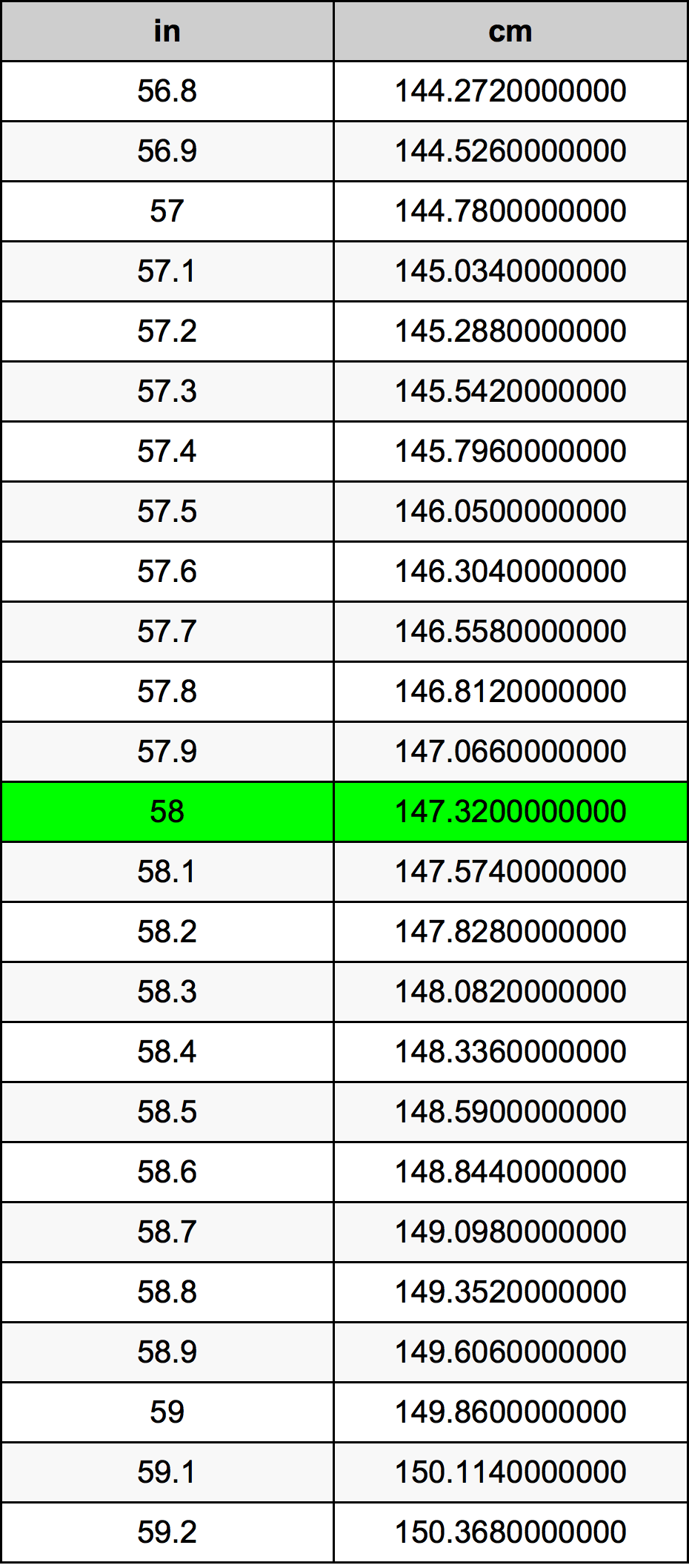Inches To Centimeters

# 58 in to cm58 Inches to Centimeters

in
=
cm

## How to convert 58 inches to centimeters?

 58 in * 2.54 cm = 147.32 cm 1 in
A common question is How many inch in 58 centimeter? And the answer is 22.8346456693 in in 58 cm. Likewise the question how many centimeter in 58 inch has the answer of 147.32 cm in 58 in.

## How much are 58 inches in centimeters?

58 inches equal 147.32 centimeters (58in = 147.32cm). Converting 58 in to cm is easy. Simply use our calculator above, or apply the formula to change the length 58 in to cm.

## Convert 58 in to common lengths

UnitLengths
Nanometer1473200000.0 nm
Micrometer1473200.0 µm
Millimeter1473.2 mm
Centimeter147.32 cm
Inch58.0 in
Foot4.8333333333 ft
Yard1.6111111111 yd
Meter1.4732 m
Kilometer0.0014732 km
Mile0.000915404 mi
Nautical mile0.0007954644 nmi

## What is 58 inches in cm?

To convert 58 in to cm multiply the length in inches by 2.54. The 58 in in cm formula is [cm] = 58 * 2.54. Thus, for 58 inches in centimeter we get 147.32 cm.

## 58 Inch Conversion Table## Alternative spelling

58 Inches to Centimeters, 58 Inches in Centimeters, 58 in to cm, 58 in in cm, 58 Inch to Centimeters, 58 Inch in Centimeters, 58 Inches to Centimeter, 58 Inches in Centimeter, 58 in to Centimeters, 58 in in Centimeters, 58 Inch to cm, 58 Inch in cm, 58 Inch to Centimeter, 58 Inch in Centimeter